# SPC/Fw model of water

(Redirected from SPC/Fw)

The SPC/Fw is a flexible variant of the rigid SPC model for water . This model has also been re-parametrised for quantum simulations, adopting the name q-SPC/Fw . The model is given by the intra-molecular component (Eq. 2 of ):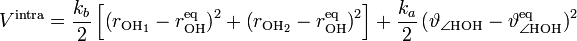$V^{\mathrm {intra}} = \frac{k_b}{2} \left[ \left( r_{\mathrm {OH}_1} - r_{\mathrm {OH}}^{\mathrm {eq}} \right)^2 + \left( r_{\mathrm {OH}_2} - r_{\mathrm {OH}}^{\mathrm {eq}} \right)^2\right] + \frac{k_a}{2} \left( \vartheta_{\angle \mathrm{HOH}} - \vartheta^{\mathrm{eq}}_{\angle \mathrm{HOH}} \right)^2$

and the inter-molecular component (Eq. 3 of ):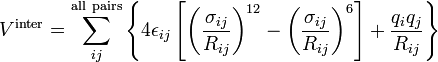$V^{\mathrm {inter}} = \sum_{ij}^{\mathrm{all~pairs}} \left\{ 4 \epsilon_{ij} \left[ \left(\frac{\sigma_{ij}}{R_{ij}} \right)^{12}- \left( \frac{\sigma_{ij}}{R_{ij}}\right)^6 \right] + \frac{q_i q_j}{R_{ij}}\right\}$

The parameters for both of these models are given in the following table (Table I of ):

 Model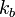$k_b$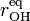$r^{\mathrm {eq}}_{\mathrm {OH}}$ (Å)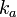$k_a$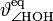$\vartheta^{\mathrm{eq}}_{\angle \mathrm{HOH}}$ (deg)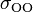$\sigma_{\mathrm {OO}}$ (Å)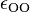$\epsilon_{\mathrm {OO}}$ (kcal mol-1) q(O) (e) q(H) (e) SPC/Fw 1059.162 1.012 75.90 113.24 3.165492 0.1554253 -0.82 0.41 q-SPC/Fw 1059.162 1.000 75.90 112.0 3.165492 0.1554252 -0.84 0.42

where the unit of$k_b$ is kcal.mol-1Å-2 and the unit of$k_a$ is kcal.mol-1rad-2.

## Dielectric constant

The dielectric constant has been calculated by Raabe and Sadus .